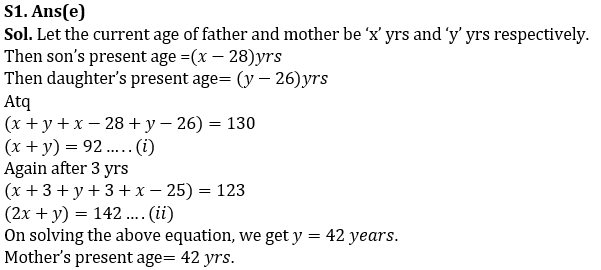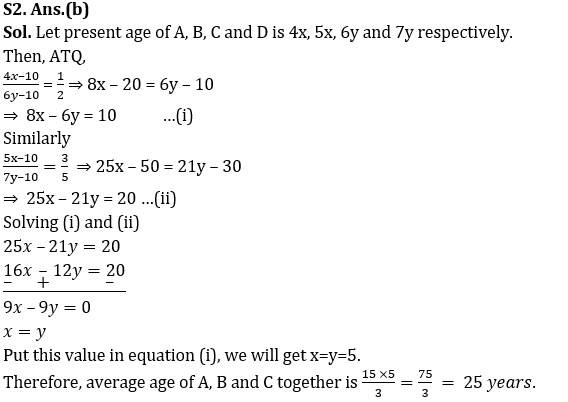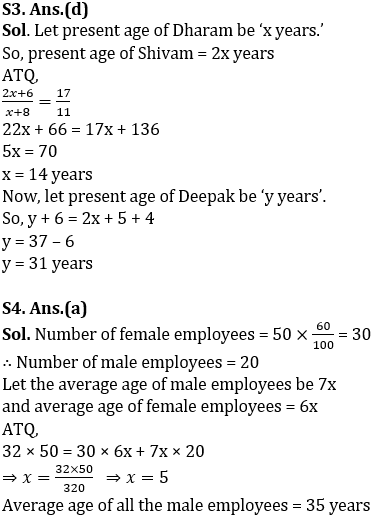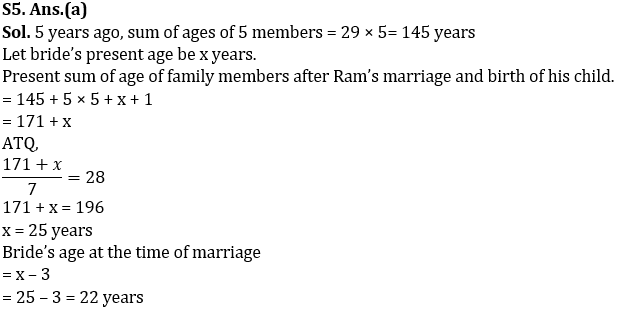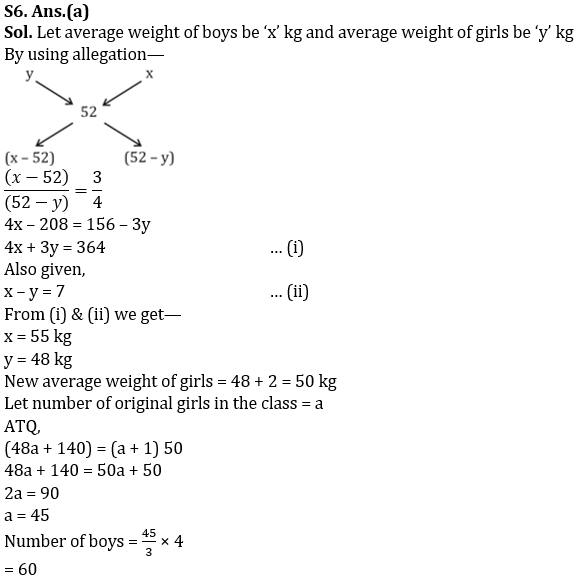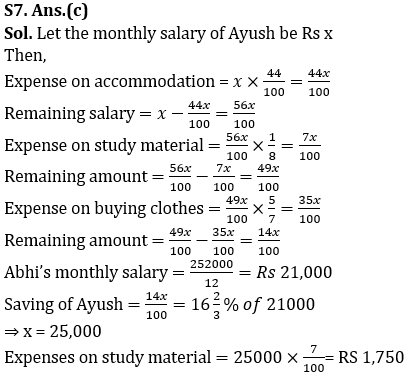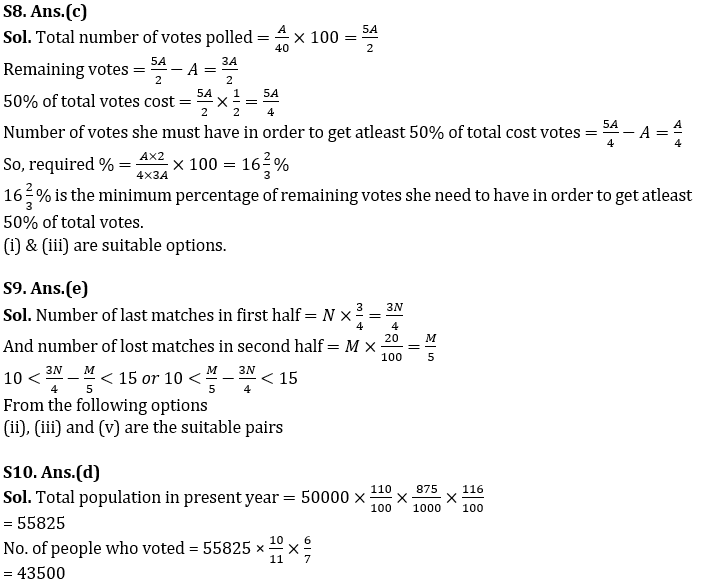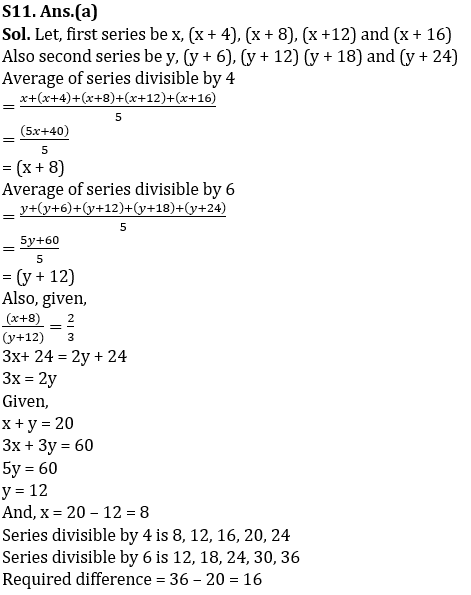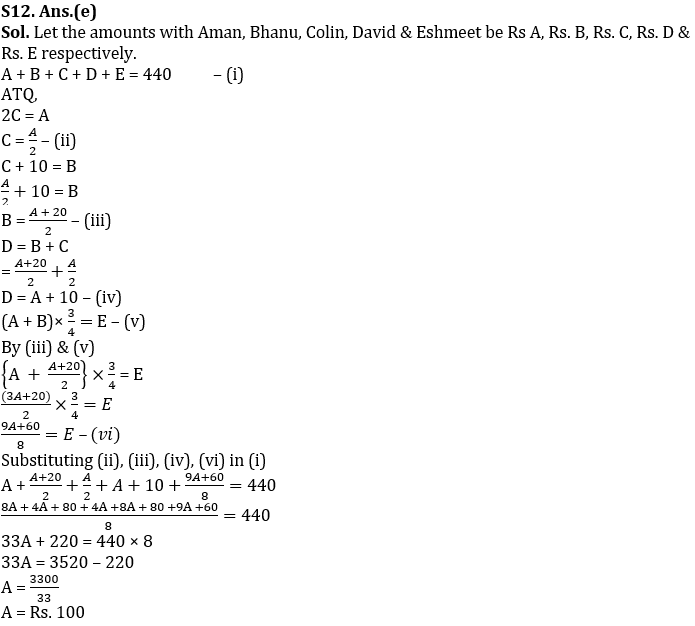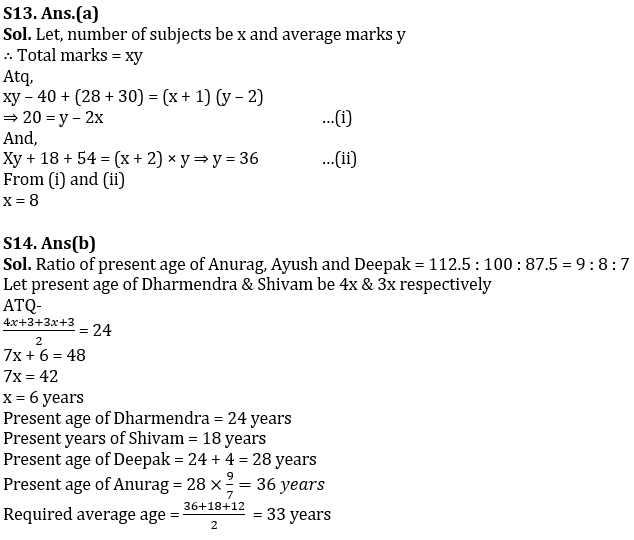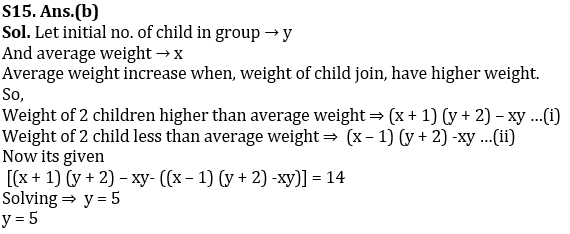# Quantitative Aptitude Quiz for IBPS 2020 Mains Exams- 27th November

Q1. My sister was born 26 yrs after my mother was born and I was born 28 yrs after my father was born. The present average age of my family is 32.5 yrs. After 3 yrs from now my sister will get married and will leave the family, then average age of family will become 41 yrs. What is the present age of my mother?(in yrs)
(a) 40
(b)50
(c) 45
(d)41
(e)42

Q2. Ratio of present age of A and B is 4 : 5, while that of C and D is 6 : 7. If 10 years ago Ratio of age of A and C is 1 : 2 and that of B & D is 3 : 5, Find average of present age of A, B and C together.
(a) 27.5 years
(b) 25 years
(c) 27 years
(d) 26.5 years
(e) 24.5 years

Q3. Age of Shivam is twice of that of Dharam. Ratio of age of Shivam 6 years hence to age of Dharam 8 years hence is 17 : 11. If age of Deepak 6 years hence will be 4 years more than the age of Shivam 5 years hence, then find the present age of Deepak.
(a) 35 years
(b) 37 years
(c) 42 years
(d) 31 years
(e) 38 years

Q4. Average age of all the employees in a company is 32 years. 60% of the employees in the company are female and the ratio of the average age of all female to average age of all male employees is 6 : 7. Find the average age of all the male employees if there are total 50 employees in the company.
(a) 35 years
(b) 42 years
(c) 28 years
(d) 49 years
(e) 30 years

Q5. Five years ago, the average age of Ram’s family having five members including Ram was 29 years. Ram got married 3 years ago and his only child was born 2 years after marriage so that present average age of family becomes 28 years. Find the age of bride at the time of marriage?
(a) 22 yrs
(b) 25 yrs
(c) 24 yrs
(d) 27 yrs
(e) 28 yrs

Q6. Average weight of a class is 52 kg and average weight of boys is 7 kg more than average weight of girls and the ratio of number of boys to girls is 4 : 3. If a girl with weight of 140 kg joined the class average weight of girls increased by 2 kg, then find the number of boys in the class?
(a) 60
(b) 72
(c) 64
(d) 36
(e) 68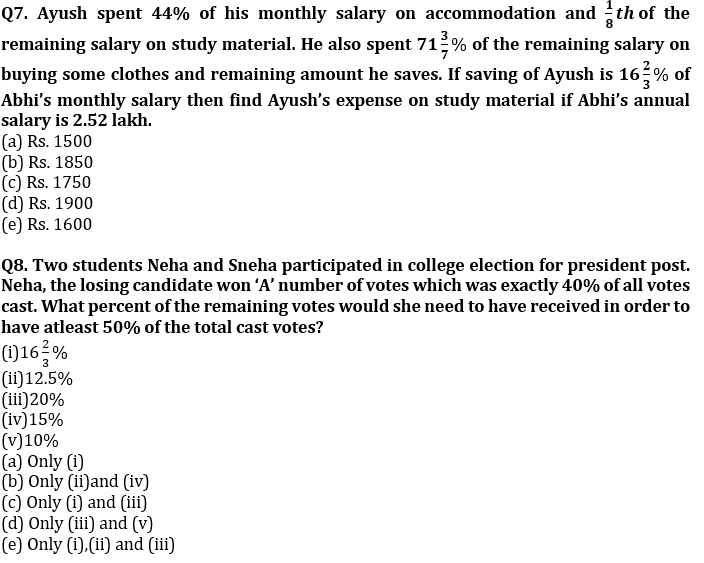Q9. A tennis player won 25% of N number of matches played in first half and won 80% of M number of matches played in second half. If the difference between number of matches lost in both halves is more than 10 but less than 15 then find which of the following pairs (N,M) of matches can be the suitable answer.(The no. of matches lost in the first half is greater than the no. of matches lost in the second half.)
(i) 44, 115
(ii)64,175
(iii) 32,50
(iv) 52,120
(v) 48,110
(a)Only (ii) and (iii)
(b)Only (i),(iii) and (v)
(c)All of the above
(d)Only (iii),(iv) and (v)
(e)None of these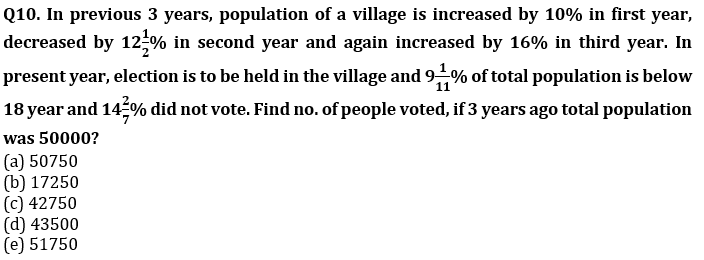Q11. The ratio of average of two series of five numbers each is 2:3. All five terms of the former series are consecutive multiples of 4 while all five terms of the latter series are consecutive multiples of 6. If sum of their first term is 20, then find the difference between greatest term of series divisible by 6 and second greatest term of series divisible by 4?
(a) 16
(b) 12
(c) 10
(d) 14
(e) 8

Q12. Aman, Bhanu, Colin, David and Eshmeet had a total of Rs.440 with them. Aman had twice the sum that Colin has. Bhanu has Rs.10 more than Colin. David’s share is equal to the sum of amount that Bhanu and Colin has, Eshmeet has 75% of the amount that Aman and Bhanu together has. Find the amount with Aman.
(a) Rs. 150
(b) Rs. 60
(c) Rs. 120
(d) Rs. 110
(e) Rs. 100

Q13. Average marks of Sandeep are decreased by 2 when he excludes 40 marks of social science and include 28 and 30 marks of physics and chemistry respectively. If he again adds 54 marks of computer science the average becomes equal to original average. Find the number of subjects originally.
(a) 8
(b) 9
(c) 10
(d) 12
(e) 15

Q14. Present age of Anurag is 12.5% more than that of Ayush and present age of Deepak is 12.5% less than that of Ayush. Ratio of present age of Dharmendra & Shivam is 4 : 3 and present age of Deepak is equal to age of Dharmendra four years hence. If average age of Dharmendra & Shivam after three years will be 24 years, then find average age of Anurag & Shivam after six years?
(a) 35 years
(b) 33 years
(c) 31 years
(d) 29 years
(e) 30 years

Q15. Average weight of group of some children increases by 1 when 2 children join them. If instead of these 2 children another 2 children join the group then average decreased by 1 but total weight becomes more than initial weight. If difference between the sums of weight of 2 children of previous one to later one is 14 then find the initial number of children in group.
(a) 7
(b) 5
(c) 3
(d) 6
(e) None of these

Practice More Questions of Quantitative Aptitude for Competitive Exams:

Solutions